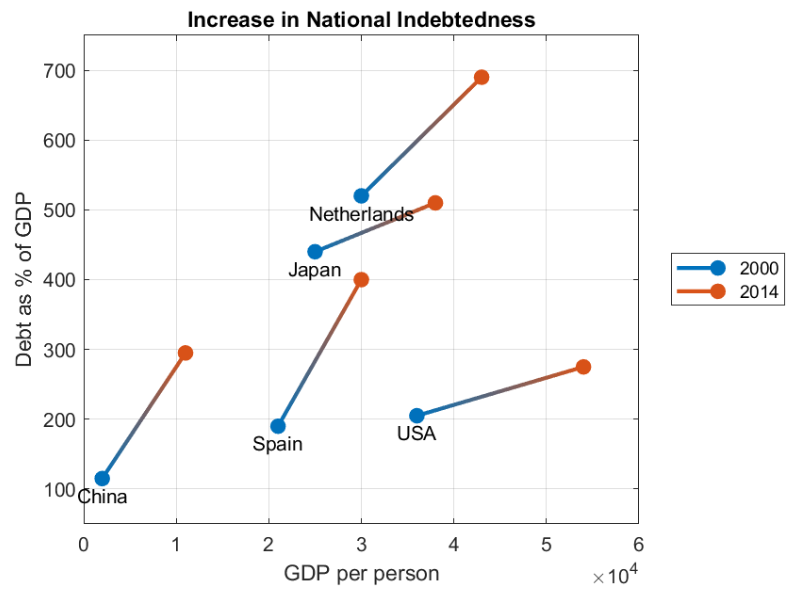## Delta Plot

버전 1.0 (147 KB) 작성자:
deltaplot creates multiple line segments, each showing the difference between pairs of values.

다운로드 수: 13

업데이트 날짜: 2021/7/13

GitHub에서 호스트

# deltaplotVersion: 1.0

deltaplot creates multiple line segments, each showing the difference between pairs of values.How to use:

``````deltaplot(X1, X2)
deltaplot(X1, Y1, X2, Y2)
deltaplot(___, names)
deltaplot(___,Name,Value)
deltaplot(Parent,___)
D = deltaplot(___)
``````

call `help deltaplot` for more information.

### 인용 양식

Abby Skofield (2022). Delta Plot (https://github.com/MATLAB-Graphics-and-App-Building/deltaplot/releases/tag/v1.0), GitHub. 검색됨 .

##### MATLAB 릴리스 호환 정보
개발 환경: R2021a
R2021a 이상 릴리스와 호환
##### 플랫폼 호환성
Windows macOS Linux

### Community Treasure Hunt

Find the treasures in MATLAB Central and discover how the community can help you!

Start Hunting!
이 GitHub 애드온의 문제를 보거나 보고하려면 GitHub 리포지토리로 가십시오.
이 GitHub 애드온의 문제를 보거나 보고하려면 GitHub 리포지토리로 가십시오.